# 复杂受限结构全聚焦超声解决方案研究The Solution Research of Complex and Restricted Structure with Total Focusing Method Ultrasonic Technology

DOI: 10.12677/APP.2021.111007, PDF, HTML, XML, 下载: 98  浏览: 138

Abstract: Fillet weld is a common welding structure in power plants. The diversity and complexity of the structure and the limitation of the probe moving space are important factors affecting the smooth implementation of ultrasonic testing. In recent years, with the development of acoustic technology and computer technology, the conventional ultrasonic testing technology and phased array ultrasonic testing technology has been widely used in the industrial test; the total focusing method ultrasound technology application in the industrial test is also increasing; but because of the own characteristics of the technology, there are some limitations for complex and restricted structure testing, for example, the inspection area cannot be fully covered by ultrasonic with limited scanning area, and the possibility of detection can’t meet the requirements. Based on the type of placement fillet weld as the breakthrough point, from multiple dimensions such as the probe design, the comparison of different ultrasonic testing methods, custom total focusing method ultrasonic algorithm research and array setting to investigate the main technical points of total focusing method ultrasonic technologies for complex and restricted structure application, we summed up a solution of complex and restricted structure with custom total focusing method ultrasonic technology.

1. 引言

$I\left(X,Z\right)={\sum }_{i=1}^{N}{\sum }_{J=1}^{N}{e}_{xi,xj}\left({t}_{i,j}\left(x,z\right)\right)$ (1)

$A\left(P\right)=|{\sum }_{q=1}^{Q}{\sum }_{j=1}^{N}{S}_{qj}\left({t}_{q}^{P}+{t}_{j}^{P}\right)|$ (2)

2. 复杂受限结构全聚焦超声成像研究方案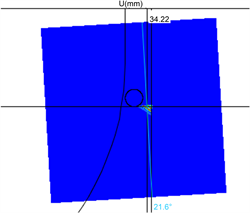(a)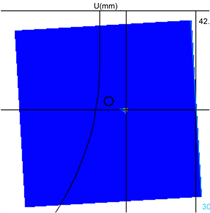(b)

Figure 1. The picture of different blow holes in branch pipe with total focusing method technology. (a) 2 mm Blow hole; (b) 1 mm Blow holeTable 1. The dimension of fillet weld block

3. 复杂受限结构标准全聚焦超声成像研究

${t}_{i}^{P}=\frac{\sqrt{{\left({x}_{in}-{x}_{i}\right)}^{2}+{z}_{in}^{2}}}{{v}_{a}}+\frac{\sqrt{{\left({x}_{r}-{x}_{in}\right)}^{2}+{\left({z}_{r}-{z}_{in}\right)}^{2}}}{{v}_{b}}+\frac{\sqrt{{\left({x}_{p}-{x}_{r}\right)}^{2}+{\left({z}_{p}-{z}_{r}\right)}^{2}}}{{v}_{c}}$ (3)

${t}_{q}^{P}=\frac{{x}_{in}\mathrm{sin}{\alpha }_{q}+{z}_{in}\mathrm{cos}{\alpha }_{q}}{{v}_{a}}+\frac{\left({x}_{r}-{x}_{in}\right)\mathrm{sin}{\beta }_{q}+\left({z}_{r}-{z}_{in}\right)\mathrm{cos}{\beta }_{q}}{{v}_{b}}+\frac{\sqrt{{\left({x}_{r}-{x}_{p}\right)}^{2}+{\left({z}_{r}-{z}_{p}\right)}^{2}}}{{v}_{c}}$ (4)

$\left({x}_{in},\text{\hspace{0.17em}}{z}_{in}\right)$ 为探头入射点的位置坐标， $\left({x}_{r},\text{\hspace{0.17em}}{z}_{r}\right)$ 为底波反射点的位置坐标， $\left({x}_{p},\text{\hspace{0.17em}}{z}_{p}\right)$ 为被检测对象的位置坐标。 ${v}_{a}$${v}_{b}$${v}_{c}$ 分别为声束在从发射到入射点、直射和一次反射时耗费的时间。 ${\alpha }_{q}$ 为声束入射到工件时的入射角， ${\beta }_{q}$ 为声束在工件底面反射时的入射角。Table 5. The test of standard total focusing method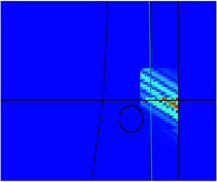(a)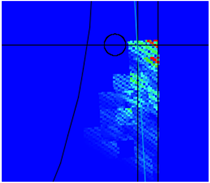(b)

Figure 2. The problem of blow hole imaging. (a) Error location; (b) Poor imaging

$API=\frac{A-6\text{\hspace{0.17em}}\text{dB}}{{\lambda }^{2}}$ (5)

4. 复杂受限结构自定义全聚焦超声成像研究Table 6. The list of custom total focusing method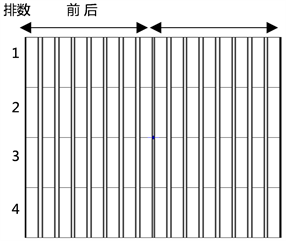Figure 3. The schematic diagram of array probe layoutTable 7. The test of custom total focusing method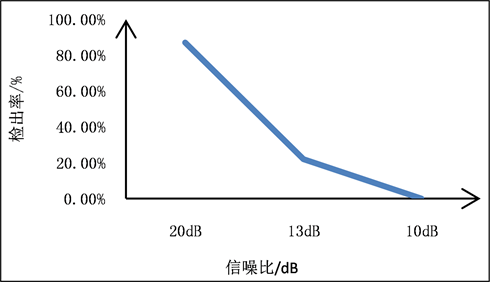Figure 4. The relationship between SNR and POD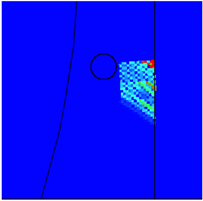(a)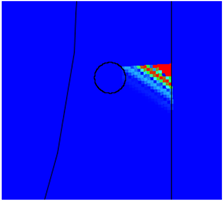(b)

Figure 5. The location accuracy of blow hole. (a) The influence of location accuracy by scattered field; (b) Normal location

5. 结论

1) 自定义全聚焦超声成像方法适合复杂受限结构特定区域微小缺陷的成像。

2) 实现复杂受限结构的全聚焦超声成像，需要使用能够实现空间立体成像的二维矩阵探头，结合自定义全聚焦成像方法，精准调节声束的偏转和传播方向。

3) 复杂受限结构自定义全聚焦超声成像方案的设计需要详细分析被检工件的结构和声束的传播路径，选择合适的全聚焦模态和模式以及全聚焦成像点的间隔。

4) 自定义全聚焦超声成像方法使用时探头的选用依然需遵循传统相控阵超声和标准全聚焦超声探头实施时探头的选用原则。

5) 信噪比和缺陷散射场是影响复杂受限结构全聚焦超声成像效果的重要因素。

6) 和标准全聚焦超声成像方法相比，自定义全聚焦成像对仪器性能有更高要求。

NOTES

*通讯作者。

  章东, 桂杰, 周哲海. 超声相控阵全聚焦无损检测技术概述[J]. 声学技术, 2018, 37(4): 320-325. http://dx.chinadoi.cn/10.16300/j.cnki.1000-3630.2018.04.005  Fan, C.G., Caleap, M., Pan, M.C. and Drinkwater, B.W. (2014) A Comparison between Ultrasonic Array Beamforming and Super Resolution Imaging Algorithms for Non-Destructive Evaluation. Ultrasonics, 54, 1842-1850. https://doi.org/10.1016/j.ultras.2013.12.012  郑驰超, 彭虎, 赵巍. 符号相干系数加权的超声平面波复合成像算法[J]. 电子学报, 2018, 46(19): 31-38. http://dx.chinadoi.cn/10.3969/j.issn.0372-2112.2018.01.005  Le Jeune, L., Robert, S., Lopez Villaverde, E. and Prada, C. (2016) Plane Wave Imaging for Ultrasonic Non-Destructive Testing: Generalization to Multimodal Imaging. Ultrasonics, 64, 128-138. https://doi.org/10.1016/j.ultras.2015.08.008  Wilcox, P.D., Holmes, C. and Drinkwater, B.W. (2007) Ad-vanced Reflector Characterization with Ultrasonic Phased Array in NDE Applications. IEEE Transaction on Ultrasonics, and Frequency Control, Ferroelectrics, and Frequency Control, 54, 1541-1550. https://doi.org/10.1109/TUFFC.2007.424  Drinkwater, B.W. and Bowler, A.I. (2009) Ultrasonic Array In-spection of Clifton Suspension Bridge Chan-Links. Insight, 51, 491-498. https://doi.org/10.1784/insi.2009.51.9.491  周正干, 彭地, 李洋, 胡宏伟. 相控阵超声检测技术中的全聚焦成像算法及其校准研究[J]. 机械工程学报, 2015, 51(10): 1-7. https://doi.org/10.3901/JME.2015.10.001  王冲, 毛捷, 廉国选. 阵元固定指向性补偿对超声全聚焦成像的优化研究[J]. 应用声学, 2018, 37(5): 732-737. http://dx.chinadoi.cn/10.11684/j.issn.1000-310X.2018.05.018  Zhang, J., Drinkwater, B.W. and Wilcox, P.D. (2010) Effects of Array Transducer Inconsistencies on Total Focusing Method Imaging Performance. NDT & E Interna-tional, 44, 361-368. https://doi.org/10.1016/j.ndteint.2011.03.001  Tasinkevych, Y., Trots, I. and Nowicki, A. (2012) Modified Synthetic Transmit Aperture Algorithm for Ultrasound Imaging. Ultrasonics, 52, 333-342. https://doi.org/10.1016/j.ultras.2011.09.003  于朋, 刚铁. 基于全聚焦成像技术的焊缝近表面平面类缺陷检测[J]. 焊接学报, 2019, 40(12): 36-40.  周进节, 郑阳, 张宗健, 谭继东. 缺陷散射对相控阵超声全聚焦超声成像影响的研究[J]. 仪器仪表学报, 2017, 38(2): 454-461.  Holmes, C., Drinkwater, B.W. and Wilcox, P.D. (2005) Post-Processing of the Full Matrix of Ultrasonic Transmit-Receive Array Data for Non-Destructive Evaluation. NDT & E International, 38, 701-711. https://doi.org/10.1016/j.ndteint.2005.04.002  贾乐成, 陈世利, 白志亮, 曾周末, 杨晓霞. 超声全聚焦成像校正模型及加速算法[J]. 仪器仪表学报, 2017, 38(7): 1589-1596.  Trots, I., Nowicki, A. and Lewandowski, M. (2009) Synthetic Transmit Aperture Method in Ultrasonic Imaging. Archives of Acoustics, 34, 685-695.